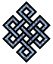#fail2ban bad ip database: ip 75.150.217.97

### | ip database | live view | stats | report | help | api key:

 ip: 75.150.217.97 hostname: 75-150-217-97-Illinois.hfc.comcastbusiness.net country:[US] United States first reported: 28.08.2018 11:58.41 GMT+0100 last reported: 03.01.2019 09:46.12 GMT+0100 time period: 127d 22h 47m 31s total reports: 12 reported by: 2 host(s) filter(s): sshd (11) ssh (1) tor exit node no badips.com db Lookup## port scan of '75.150.217.97':

[-hide]
```# Nmap 6.40 scan initiated Tue Aug 28 11:59:01 2018 as: /usr/bin/nmap -sU -sS -O 75.150.217.97
Nmap scan report for 75-150-217-97-Illinois.hfc.comcastbusiness.net (75.150.217.97)
Host is up (0.11s latency).
Not shown: 999 open|filtered ports, 992 closed ports
PORT     STATE    SERVICE
22/tcp   open     ssh
80/tcp   open     http
135/tcp  filtered msrpc
139/tcp  filtered netbios-ssn
445/tcp  filtered microsoft-ds
1723/tcp open     pptp
8080/tcp open     http-proxy
8383/tcp open     m2mservices
500/udp  open     isakmp
No exact OS matches for host (If you know what OS is running on it, see http://nmap.org/submit/ ).
TCP/IP fingerprint:
OS:SCAN(V=6.40%E=4%D=8/28%OT=22%CT=1%CU=%PV=N%G=Y%TM=5B851E56%P=x86_64-pc-l
OS:inux-gnu)SEQ(SP=107%GCD=1%ISR=10A%TI=Z%CI=Z%TS=U)OPS(O1=M5B4NNSNW7%O2=M5
OS:78NNSNW7%O3=M280NW7%O4=M22CNNSNW7%O5=M218NNSNW7%O6=M109NNS)WIN(W1=3908%W
OS:2=3908%W3=3908%W4=3908%W5=3908%W6=3908)ECN(R=Y%DF=Y%TG=40%W=3908%O=M5B4N
OS:NSNW7%CC=Y%Q=)T1(R=Y%DF=Y%TG=40%S=O%A=S+%F=AS%RD=0%Q=)T2(R=N)T3(R=N)T4(R
OS:=Y%DF=Y%TG=40%W=0%S=A%A=Z%F=R%O=%RD=0%Q=)T5(R=Y%DF=Y%TG=40%W=0%S=Z%A=S+%
OS:F=AR%O=%RD=0%Q=)T6(R=Y%DF=Y%TG=40%W=0%S=A%A=Z%F=R%O=%RD=0%Q=)T7(R=N)U1(R
OS:=N)IE(R=N)

OS detection performed. Please report any incorrect results at http://nmap.org/submit/ .
# Nmap done at Tue Aug 28 12:05:10 2018 -- 1 IP address (1 host up) scanned in 369.34 seconds
```
```Σ = 41 | Δt = 0.0034530162811279s
```